### 2.2.2.1 Drift Current

The component of the current which is caused by the electric field is called drift current. From the macroscopic point of view the current density and the electric field are related by OHM's law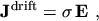(2.20)

where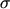is the electrical conductivity. It is in general a tensor but can be assumed as a scalar in most semiconductors2.3.

From the microscopic point of view the current density is made up by the moving carriers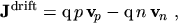(2.21)

where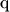is the elementary charge,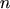and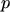are the electron and hole concentrations, respectively, and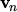and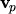are the mean velocities of electrons and holes, respectively. The electric field accelerates the carriers, but due to various scattering mechanisms the velocity of the carriers is limited: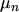(2.22)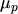(2.23)

whereandare the mobility of electrons and holes, respectively. By inserting these two equations into eqn. (2.21)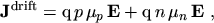(2.24)

and comparing eqn. (2.24) with eqn. (2.20) and eqn. (2.19) the following relations are obtained: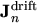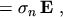(2.25)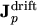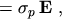(2.26)

and for the conductivities: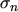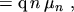(2.27)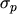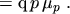(2.28)

M. Gritsch: Numerical Modeling of Silicon-on-Insulator MOSFETs PDF